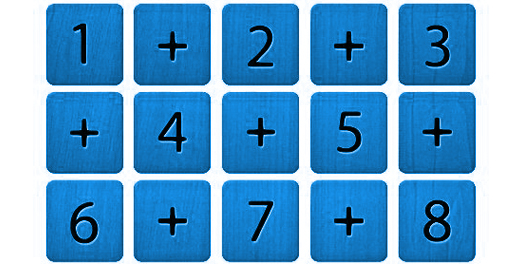Our Address: FZ LLC G 37 & G 34, Block 13, Al Sufouh Road Knowledge Park, Dubai PO Box : 501703

# Odd and EvenAll integers fall into either one of the two categories – odd or even. Odd numbers are those which are not divisible by 2 while even numbers are divisible by 2.

Odds: -5, -3, -1, 1, 3, 5…

Evens: -4, -2, 0, 2, 4…

Consecutive integers alternate between odd and even. E.g. 1 (odd), 2 (even), 3 (odd), 4 (even)…

Combining Odds and Evens

The GMAT tests your knowledge of how odd and even numbers combine through addition, subtraction, multiplication, and division. The following rules would come in handy.

If you add or subtract same type (both odd or both even), the answer is even. E.g. 3(odd) + 5(odd) = 8(even), 8(even)-2(even) = 6(even).

If you add or subtract different types (one odd and other even), the answer is odd. E.g. 3(odd) +6(even) = 9(odd)

Multiplication

When you multiply integers, if ANY of the integers is even, the result is EVEN. E.g. 5 x 3 x 2 = 30

Likewise, if NONE of the integers is even, then the result is ODD. E.g. 5 x 7 x 9 = 315

To summarize so far:

Odd ± Even = ODD Odd x Odd = ODD

Odd + Odd = EVEN Even x Even = EVEN (and divisible by 4)

Even ± Even = EVEN Odd x Even = EVEN

Representing Evens and Odd Algebraically

Odd numbers are one more or one less than multiples of 2, so any odd number can be written as 2n + 1 or 2n – 1, where n is an integer. Even numbers are multiples of 2, so any even number can be written as 2n, where n is any integer.

Such representation is useful when the only thing known about an integer is that it is odd or even. E.g.

What is the remainder when k is divided by 4?

(1) k is the square of an odd integer.

(2) k is a multiple of 3.

One way to evaluate Statement (1) is to square a series of odd integers, divide each of the squares by 4, and observe the remainders. However, we can use the representation of an odd integer to solve the problem faster. Any odd integer can be written as 2n + 1, where n is an integer. Therefore, the square of any odd integer can be written as (2n + l)2 = 4n2 + 4n + 1. The first two terms of this expression are multiples of 4, which have remainder 0 when divided by 4. The third term, 1, gives a remainder of 1 when divided by 4 (1/4 = 0 with remainder 1). So the overall expression must have remainder 1 when divided by 4. Thus, (1) is SUFFICIENT.

(2) can be proven insufficient by taking examples. When 6 is divided by 4, the remainder is

2; when 9 is divided by 4, the remainder is 1. Since we get two different answers, (2) is INSUFFICIENT. Hence the correct answer is (A).

It is advisable to do a lot of practice on odds and evens as the topic is very frequently asked.

At Option Training Institute, our approach is to focus on problem solving so that you go into the test fully capable of handling anything that GMAT throws at you.